Next: Isothermal Atmosphere Up: Classical Thermodynamics Previous: Isothermal and Adiabatic Expansion

# Hydrostatic Equilibrium of Atmosphere

The gas that we are most familiar with in everyday life is, of course, the Earth's atmosphere. It turns out that we can use the isothermal and adiabatic gas laws to explain most of the observed features of the atmosphere.

Let us, first of all, consider the hydrostatic equilibrium of the atmosphere. Consider a thin vertical slice of the atmosphere, of cross-sectional area, that starts at heightabove ground level, and extends to height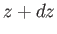. The upward force exerted on this slice by the gas below it is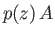, where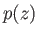is the pressure at height. Likewise, the downward force exerted by the gas above the slice is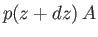. The net upward force is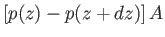. In equilibrium, this upward force must be balanced by the downward force due to the weight of the slice, which is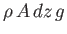, where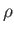is the mass density of the gas, and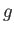the acceleration due to gravity. It follows that the force balance condition can be written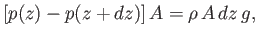(6.63)

which reduces to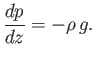(6.64)

This result is known as the equation of hydrostatic equilibrium for the atmosphere.

We can express the mass density of a gas in the following form,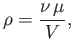(6.65)

where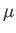is the molecular weight of the gas, and is equal to the mass of one mole of gas particles. For instance, the molecular weight of nitrogen gas is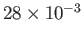kg. The previous formula for the mass density of a gas, combined with the ideal gas law,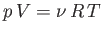, yields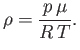(6.66)

It follows that the equation of hydrostatic equilibrium can be rewritten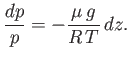(6.67)Next: Isothermal Atmosphere Up: Classical Thermodynamics Previous: Isothermal and Adiabatic Expansion
Richard Fitzpatrick 2016-01-25